# 2021年成考高起点文科数学真题及答案

## 试卷预览

• 1.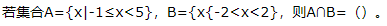A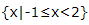B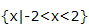C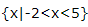D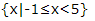• 2. 已知函数f(x)=ax3.若f'(3)=9，则a=（）。

A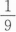B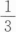C1

D3

• 3. 若向量a=(3，4)，则与a方向相同的单位向量为（）。

A(0，1)

B(1，0)

C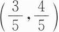D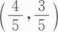• 4. 过抛物线C：y2=4x的焦点作2轴的垂线，交C于A，B两点，则|AB|=（）。

A2

B4

C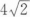D8

• 5.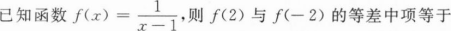A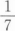B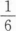C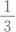D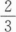• 1. 若28，37，x，30四个数的平均数为35，则x=（）。
• 2. 圆X2+y2=5在点(1，2)处切线的方程为（）。
• 3. 已知函数f(x)=2x+1，则f(2x)=（）。
• 4.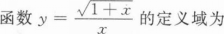• 1. 已知A，B为⊙O上的两点，且AB=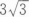∠ABO=30°.求⊙O的半径。
• 2. 已知{an}是公差不为0的等差数列，且a2，a6，a12成等比数列，a2+a6+a12=76.求{an}的通项公式。
• 3. 已知函数f(x)=2x3—3x2+2。(I)求f'(x)；
(Ⅱ)求f(x)在区间[一2，2]的最大值与最小值
• 4.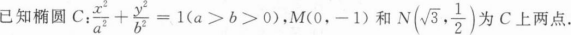(Ⅰ)求C的标准方程；
(Ⅱ)求C的左焦点到直线MN的距离